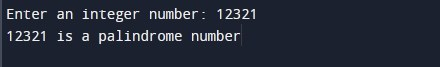# C Program to Check Palindrome Number

In this tutorial you will learn about the C Program to Check Palindrome Number and its application with practical example.

In this tutorial, we will learn to create a C Program to check palindrome number using C programming language. In this article we will create C programs to check if the input number is palindrome or not. 1) using while loop 2) using function 3) using recursion.

Prerequisites

Before starting with this tutorial we assume that you are best aware of the following C programming topics:

• C if else
• C while loop
• C do while loop
• C Function
• C Recursion

## What Is Palindrome Number?

A palindrome number is a number that remains the same even when its digits are reversed. For example 121, 212, 12321 are palindrome numbers. Let’s take example of 12321, when we reverse its digits we get 12321 that is equal to original number.

## C Program to Check Palindrome Number Using While Loop

In this program we will check palindrome number using while loop. We would first declared and initialized the required variables. Next, we would prompt user to input an integer number. Later in the program we will to check whether given number is palindrome or not using while loop. Finally, we will display given number is palindrome or not.

Output:-In the above program, we have first declared and initialized a set variables required in the program.

• num = it hold the input number
• rnum = used to hold the reverse number
• rem = it hold remainder
• temp = it hold temporary value

In the next statement user will be prompted to enter the an integer number value which will be assigned to variable ‘num’ . Then, we will loop through the input number to find reverse of the number and assign it to ‘rnum’. We will now compare ‘rnum’ and ‘num’ as following:

if it return true, we will display input number is a palindrome number. If it return false, we will display input number is not a palindrome number.

## Check Palindrome Number Using Function

In this program we will check palindrome number by creating a user defined function. We would first declared and initialized the required variables. Next, we would prompt user to input an integer number. Later in the program we will to check whether given number is palindrome or not using a function. Finally, we will display given number is palindrome or not.

Output:-In the above program, we have first defined following function:

checkPalindrome() :- This function means to accept an integer number and return true(1) or false(0) value.

Next, we have declared and initialized a set variables required in the program.

• num = it holds the first integer number value

In the next statement user will be prompted to enter the an integer number value which will be assigned to variable ‘num’ . Then, we will call checkPalindrome() function to find and compare reverse of the number with original number. This will return true(1) or false(0) value.

We will now compare return value of checkPalindrome() function as following:

if the above condition return true, we will display input number is a palindrome number. If it return false, we will display input number is not a palindrome number.

## Check Palindrome Number Using Recursion

In this program we will check palindrome number by creating a recursive function. We would first declared and initialized the required variables. Next, we would prompt user to input an integer number. Later in the program we will to check whether given number is palindrome or not using a recursive function. Finally, we will display given number is palindrome or not.

Output:-In the above program, we have first defined a recursive function called as checkPalindrome() that will recursively compute the reverse of the given number. Later in the main function, we declared required variables.

• num = it holds a integer number value

In the next statement user will be prompted to enter an integer number which will be assigned to variable ‘num’. Next, we will be calling checkPalindrome() function to return reverse number of the input number, We will assign reverse number to ‘rnum’. We will now compare ‘rnum’ and ‘num’ as following:

if it return true, we will display input number is a palindrome number. If it return false, we will display input number is not a palindrome number.

In this tutorial we have learn about the C Program to Check Palindrome Number and its application with practical example. I hope you will like this tutorial.